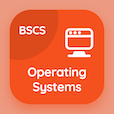Computer Science Courses Online

Digital Logic Design Quiz Questions

Digital Logic Design Practice Test 43

# Introduction to Combinational Logics Quiz Answers PDF - 43

The Book Introduction to Combinational Logics Quiz Questions and Answers PDF, introduction to combinational logics Quizzes, download chapter 6-43 to download online digital logic design course. Study Combinational Logics MCQ Questions PDF, introduction to combinational logics Multiple Choice Questions (MCQ Quiz) for online college degrees. The eBook Introduction to Combinational Logics Quiz App Download: introduction to combinational logics, introduction to integrated circuit, lamp handball, magnitude comparator, binary numbers test prep for computer software engineer.

The Quiz: Variable in Boolean expressions can be expressed as PDF, "Introduction to Combinational Logics Quiz" App (Android & iOS) Free with unprimed, primed, even, and both a and b choices for computer science programs. Practice combinational logics questions and answers, Google eBook to download free sample for cheapest online computer science degree.

## Logic Design: Introduction to Combinational Logics MCQs Quiz

MCQ: Variable in Boolean expressions can be expressed as

A) primed
B) unprimed
C) even
D) both a and b

MCQ: RTL stands for

A) Resistor Transistor Logic
B) Resistor Transistor League
C) Resistance Transistor Logic
D) Resistor Transmission Logic

MCQ: To start the game in lamp handball the start switch start the at the

A) extreme left side
B) extreme right side
C) both a and b
D) middle

MCQ: One that is not the outcome of magnitude comparator is

A) a>b
B) a-b
C) a
D) a=b

MCQ: The digital number system has a base and radix of

A) 6
B) 10
C) 2
D) 0

### Mock Tests: Digital Logic Design Course Prep

Download Digital Logic Design Quiz App, Operating Systems MCQ App, and DBMS MCQs App to install for Android & iOS devices. These Apps include complete analytics of real time attempts with interactive assessments. Download Play Store & App Store Apps & Enjoy 100% functionality with subscriptions!